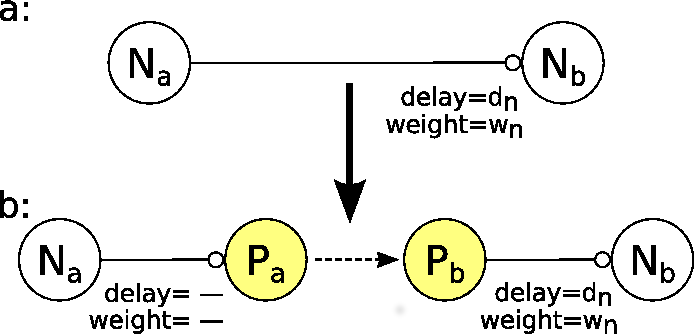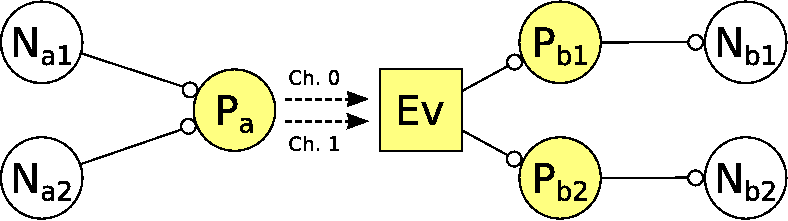# Introduction to the MUSIC Interface¶

The MUSIC interface, a standard by the INCF, allows the transmission of data between applications at runtime. It can be used to couple NEST with other simulators, with applications for stimulus generation and data analysis and visualization and with custom applications that also use the MUSIC interface.

Note

Please note that MUSIC and the recording backend for Arbor are mutually exclusive and cannot be enabled at the same time.

## Setup of System¶

To use MUSIC with NEST, we first need to ensure MUSIC is installed on our system and NEST is configured properly.

Please install MUSIC using the instructions on the MUSIC website.

In the installation of NEST, you need to add the following configuration option to your CMake.

cmake -Dwith-music=[ON </path/to/music>]
make
make install


## A Quick Introduction to NEST and MUSIC¶

In this tutorial, we will show you how to use the MUSIC library together with NEST. We will cover how to use the library from PyNEST and from the SLI language interface. In addition, we’ll introduce the use of MUSIC in a C++ application and how to connect such an application to a NEST simulation.

Our aim is to show practical examples of how to use MUSIC, and highlight common pitfalls that can trip the unwary. Also, we assume only a minimal knowledge of Python, C++ and (especially) SLI, so the examples will favour clarity and simplicity over elegance and idiomatic constructs.

While the focus here is on MUSIC, we need to know a few things about how NEST works in order to understand how MUSIC interacts with it.

### The Basics of NEST¶

A NEST network consists of three types of elements: neurons, devices, and connections between them.

Neurons are the basic building blocks, and in NEST they are generally spiking point neuron models. Devices are supporting units that for instance generate inputs to neurons or record data from them. The Poisson spike generator, the spike recorder recording device, and the MUSIC input and output proxies are all devices. Neurons and devices are collectively called nodes, and are connected using connections.

Connections are unidirectional and carry events between nodes. Each neuron can get multiple input connections from any number of other neurons. Neuron connections typically carry spike events, but other kinds of events, such as voltages and currents, are also available for recording devices. Synapses are not independent nodes, but are part of the connection. Synapse models will typically modify the weight or timing of the spike sent on to the neuron. All connections have a synapse, by default the static_synapse.Figure 31 A: Two connected neurons $$N_a$$ and $$N_b$$, with a synapse $$S$$ and a receptor $$R$$. A spike with weight $$W_a$$ is generated at $$t_0$$. B: The spike traverses the synapse and is added to the queue in the receptor. C: The receptor processes the spike at time $$t_0 + d$$.

Connections have a delay and a weight. All connections are implemented on the receiving side, and the interpretation of the parameters is ultimately up to the receiving node. In Figure 31 A, neuron $$N_a$$ has sent a spike to $$N_b$$ at time $$t$$, over a connection with weight $$w_a$$ and delay $$d$$. The spike is sent through the synapse, then buffered on the receiving side until $$t+d$$ (Figure 31 B). At that time it’s handed over to the neuron model receptor that converts the spike event to a current and applies it to the neuron model (Figure 31 C).

In NEST you use MUSIC with a pair of extra devices called proxies that create a MUSIC connection between them across simulations. The pair effectively works just like a regular connection within a single simulation. Each connection between MUSIC proxies is called a port, and connected by name in the MUSIC configuration file.

Each MUSIC port can carry multiple numbered channels. The channel is the smallest unit of transmission, in that you can distinguish data flowing in different channels, but not within a single channel. Depending on the application a port may have one or many channels, and a single channel can carry the events from one single neuron model or the aggregate output of many neurons.Figure 32 A: Two connected neurons $$N_a$$ and $$N_b$$, with delay $$d_n$$ and weight $$w_n$$. B: We’ve added a MUSIC connection with an output proxy $$P_a$$ on one end, and an input proxy $$P_b$$ on the other.

In Figure 32 A we see a regular NEST connection between two neurons $$N_a$$ and $$N_b$$. The connection carries a weight $$w_n$$ and a delay $$d_n$$. In Figure 32 B we have inserted a pair of MUSIC proxies into the connection, with an output proxy $$P_a$$ on one end, and input proxy $$P_b$$ on the other.

As we mentioned above, MUSIC proxies are devices, not regular neuron models. Like most devices, proxies ignore weight and delay parameters on incoming connections. Any delay applied to the connection from $$N_a$$ to the output proxy $$P_a$$ is thus silently ignored. MUSIC makes the inter-simulation transmission delays invisible to the models themselves, so the connection from $$P_a$$ to $$P_b$$ is effectively zero. The total delay and weight of the connection from $$N_a$$ to $$N_b$$ is thus that set on the $$P_b$$ to $$N_b$$ connection.Figure 33 A MUSIC connection with two outputs and two inputs. A single output proxy sends two channels of data to an input event handler that divides the channels to the two input proxies. They connect the recipient neuron models.

When we have multiple channels, the structure looks something like in Figure 33. Now we have two neurons $$N_{a1}$$ and $$N_{a2}$$ that we want to connect to $$N_{b1}$$ and $$N_{b2}$$ respectively. As we mentioned above, NEST devices can accept connections from multiple separate devices, so we only need one output proxy $$P_a$$. We connect each input to a different channel.

Nodes can only output one connection stream, so on the receiving side we need one input proxy $$P_b$$ per input. Internally, there is a single MUSIC event handler device $$Ev$$ that accepts all inputs from a port, then sends the appropriate channel inputs to each input proxy. These proxies each connect to the recipient neurons as above.

## Publication¶

Djurfeldt M. et al. 2010. Run-time interoperability between neuronal network simulators based on the music framework. Neuroinformatics. 8(1):43–60. DOI: 10.1007/s12021-010-9064-z.